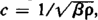# Densimeter

Also found in: Dictionary, Thesaurus, Medical, Legal, Wikipedia.
Related to Densimeter: densitometer

## densimeter

[den′sim·əd·ər]
(engineering)
An instrument which measures the density or specific gravity of a liquid, gas, or solid. Also known as densitometer; density gage; density indicator; gravitometer.
McGraw-Hill Dictionary of Scientific & Technical Terms, 6E, Copyright © 2003 by The McGraw-Hill Companies, Inc.
The following article is from The Great Soviet Encyclopedia (1979). It might be outdated or ideologically biased.

## Densimeter

an instrument for continuous or batch measurement of the density of substances during their production or processing; built into production lines or equipment. Densimeters for measuring the density of liquids, which are the most common, are divided into float, balance, hydrostatic, radioisotope, vibration, and ultrasonic types, according to their principle of operation. A group of devices for measuring the concentrations of solutions—alcoholometers, saccharometers, oil pycnometers, and lactometers for measuring the fat content of milk—are similar to densimeters.

Float densimeters are constructed with a free float, which is a constant-mass hydrometer, or with a submerged float, which is a constant-volume hydrometer. The error of densimeters of this group is ±(0.2–2) percent of the range of measurement, depending on the design.

Balance densimeters are based on the continuous weighing of a certain volume of liquid. The error of such densimeters is ±(0.5–1) percent. In hydrostatic densimeters, the difference Δρ in the pressures of two columns of liquid of different heights is a measure of the density ρ:Δp = ρgh, where g is the acceleration due to gravity and h is the difference in height of the columns. The value of Δρ is measured directly by pressure sensors or as the difference in pressures required to force out bubbles of a gas (air) in the liquid at various depths. The error of such densimeters reaches ±(2–4) percent of the range of measurement.

The operation of radioisotope densimeters is based on measurement of the change in intensity of a beam of gamma or beta rays as a result of their absorption or dispersion by a layer of liquid (the attenuation of the beam for a given thickness of the layer is determined by the density of the liquid). The error of radioisotope densimeters is of the order of 2 percent of the range of measurement.

The sensor of a vibration densimeter contains a body (a hollow cylinder, a plate, or a tuning fork) to which vibrations are imparted from without. The resonance frequency of the vibrations of the body in the substance is determined; the frequency decreases with increasing density of the substance tested. The error of vibration densimeters is ±(1–2) × 10-4 g/cm3.

The operation of an ultrasonic densimeter is based on the dependence of the speed of sound c in a medium on the density of the medium:where β is the coefficient of adiabatic compressibility of the liquid. The error of such densimeters is about 2-5 percent of the range of measurement.

Radioisotope, ultrasonic, vibration, and a number of other methods may be used to determine the density of solids and gases.

### REFERENCES

Kivilis, S. Sh. “Pribory dlia izmereniia plotnosti zhidkostei i gazov.” In Priborostroenie i sredstva avtomatiki, vol. 2, book 2. Moscow, 1964.
Izmerenie massy, ob”ema i plotnosti Moscow, 1972.
Glybin, I. P. Avtomaticheskie plotnomery. Kiev, 1965.

S. SH. KIVILIS

## densitometer

i. An instrument for measuring the density or specific gravity of fuel. Also called a densimeter.
ii. An instrument for measuring the optical density (photographic transmission, photographic reflection, visual transmission, etc.) of a material, generally of a photographic image.